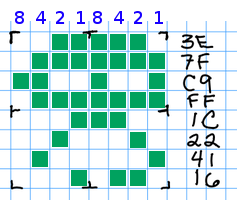# Quiz 2 Solutions

[8 points for showing up]

You have up to 25 minutes. You may use a calculator, but no text book or notes.

1. Use the hexadecimal codes in the right column to fill in the 8×8 icon grid, assuming 1 bit per pixel. [8 points]Note that I probably intended `36` on the last row… it looks like there’s a piece missing. This is indeed from Space Invaders.

2. If an image uses 6 bits per pixel, what is the maximum number of colors it can contain? [8 points]

With 6 bits per pixel, we can have 2⁶ colors, and 2⁶ = 64.

3. Suppose you work for a CPU manufacturer that has exhausted its supply of XOR gates. Show whether or not the expressionis equivalent toby completing a truth table of all possible values of inputsand. [8 points]

They are the same. Below is the truth table that proves it. You do not need to write the circuit diagram shown above the table – that’s just a convenient way to show how each column is calculated.Truth table

Recall that XOR is a way to say “not equal to.” Its result is true when A and B are different.

4. In the memory hierarchy, what is the main difference between volatile and persistent memory? [8 points]

Some of you just stated the main organizing principle of the memory hierarchy: faster, smaller capacity vs. slower, larger capacity. That may be true, but the main difference is what happens when the power is cut.

Volatile memory loses its content, while persistent memory retains it.